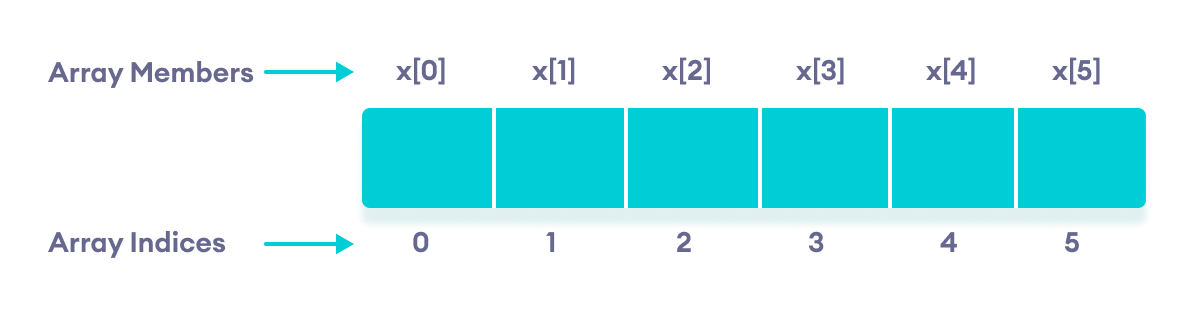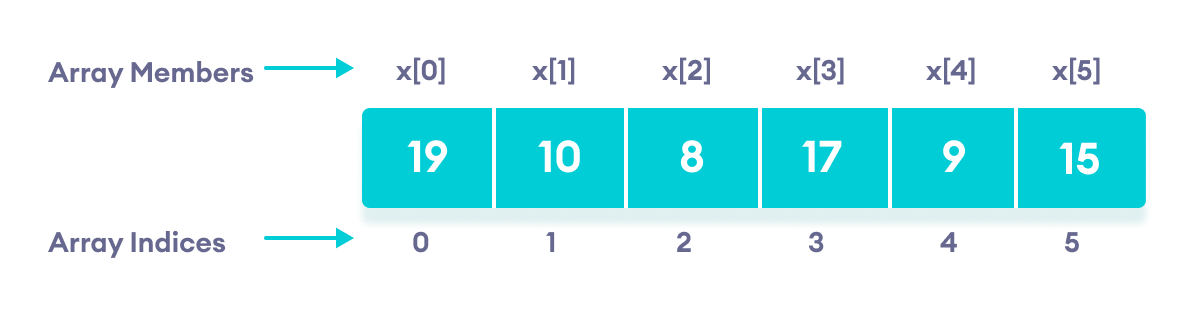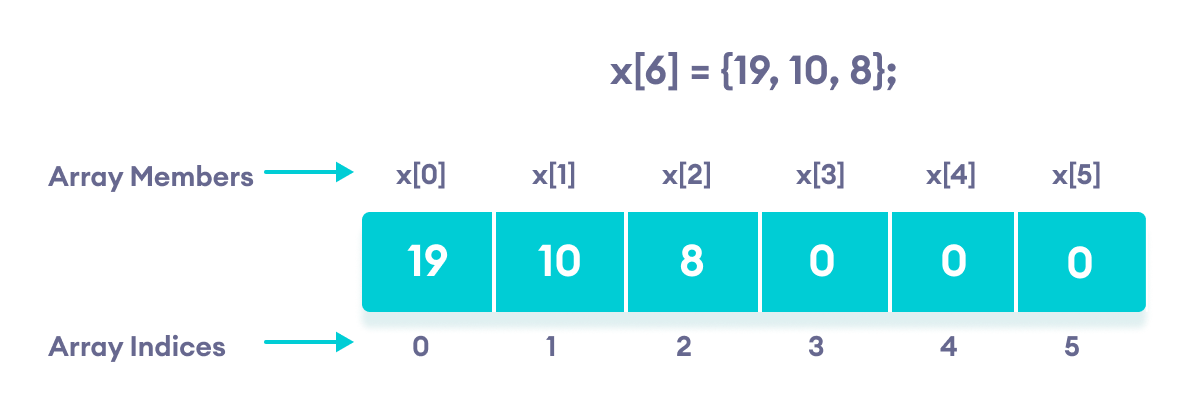# C++ Arrays

In this tutorial, we will learn to work with arrays. We will learn to declare, initialize, and access array elements in C++ programming with the help of examples.

In C++, an array is a variable that can store multiple values of the same type. For example,

Suppose a class has 27 students, and we need to store the grades of all of them. Instead of creating 27 separate variables, we can simply create an array:

``double grade;``

Here, grade is an array that can hold a maximum of 27 elements of `double` type.

In C++, the size and type of arrays cannot be changed after its declaration.

## C++ Array Declaration

``dataType arrayName[arraySize];``

For example,

``int x;``

Here,

• `int` - type of element to be stored
• x - name of the array
• `6` - size of the array

## Access Elements in C++ Array

In C++, each element in an array is associated with a number. The number is known as an array index. We can access elements of an array by using those indices.

``````// syntax to access array elements
array[index];``````

Consider the array x we have seen above.Elements of an array in C++

### Few Things to Remember:

• The array indices start with `0`. Meaning x is the first element stored at index `0`.
• If the size of an array is `n`, the last element is stored at index `(n-1)`. In this example, x is the last element.
• Elements of an array have consecutive addresses. For example, suppose the starting address of `x` is 2120.

Then, the address of the next element `x` will be 2124, the address of `x` will be 2128, and so on.

Here, the size of each element is increased by 4. This is because the size of `int` is 4 bytes.

## C++ Array Initialization

In C++, it's possible to initialize an array during declaration. For example,

``````// declare and initialize and array
int x = {19, 10, 8, 17, 9, 15};``````C++ Array elements and their data

Another method to initialize array during declaration:

``````// declare and initialize an array
int x[] = {19, 10, 8, 17, 9, 15};``````

Here, we have not mentioned the size of the array. In such cases, the compiler automatically computes the size.

### C++ Array With Empty Members

In C++, if an array has a size `n`, we can store upto n number of elements in the array. However, what will happen if we store less than n number of elements.

For example,

``````// store only 3 elements in the array
int x = {19, 10, 8};``````

Here, the array x has a size of `6`. However, we have initialized it with only 3 elements.

In such cases, the compiler assigns random values to the remaining places. Oftentimes, this random value is simply `0`.Empty array members are automatically assigned the value 0

## How to insert and print array elements?

``````int mark = {19, 10, 8, 17, 9}

// change 4th element to 9
mark = 9;

// take input from the user
// store the value at third position
cin >> mark;

// take input from the user
// insert at ith position
cin >> mark[i-1];

// print first element of the array
cout << mark;

// print ith element of the array
cout >> mark[i-1];``````

## Example 1: Displaying Array Elements

``````#include <iostream>
using namespace std;

int main() {

int numbers = {7, 5, 6, 12, 35};

cout << "The numbers are: ";

//  Printing array elements
// using range based for loop
for (const int &n : numbers) {
cout << n << "  ";
}

cout << "\nThe numbers are: ";

//  Printing array elements
// using traditional for loop
for (int i = 0; i < 5; ++i) {
cout << numbers[i] << "  ";
}

return 0;
}``````

Output

```The numbers are: 7  5  6  12  35
The numbers are: 7  5  6  12  35```

Here, we have used a `for` loop to iterate from `i = 0` to `i = 4`. In each iteration, we have printed `numbers[i]`.

We again used a range-based `for` loop to print out the elements of the array. To learn more about this loop, check C++ Ranged for Loop.

Note: In our range-based loop, we have used the code `const int &n` instead of `int n` as the range declaration. However, the `const int &n` is more preferred because:

1. Using `int n` simply copies the array elements to the variable n during each iteration. This is not memory-efficient.

&n, however, uses the memory address of the array elements to access their data without copying them to a new variable. This is memory-efficient.
2. We are simply printing the array elements, not modifying them. Therefore, we use `const` so as not to accidentally change the values of the array.

## Example 2: Take Inputs from User and Store Them in an Array

``````#include <iostream>
using namespace std;

int main() {

int numbers;

cout << "Enter 5 numbers: " << endl;

//  store input from user to array
for (int i = 0; i < 5; ++i) {
cin >> numbers[i];
}

cout << "The numbers are: ";

//  print array elements
for (int n = 0; n < 5; ++n) {
cout << numbers[n] << "  ";
}

return 0;
}``````

Output

```Enter 5 numbers:
11
12
13
14
15
The numbers are: 11  12  13  14  15```

Once again, we have used a `for` loop to iterate from `i = 0` to `i = 4`. In each iteration, we took an input from the user and stored it in `numbers[i]`.

Then, we used another `for` loop to print all the array elements.

## Example 3: Display Sum and Average of Array Elements Using for Loop

``````#include <iostream>
using namespace std;

int main() {

// initialize an array without specifying size
double numbers[] = {7, 5, 6, 12, 35, 27};

double sum = 0;
double count = 0;
double average;

cout << "The numbers are: ";

//  print array elements
// use of range-based for loop
for (const double &n : numbers) {
cout << n << "  ";

//  calculate the sum
sum += n;

// count the no. of array elements
++count;
}

// print the sum
cout << "\nTheir Sum = " << sum << endl;

// find the average
average = sum / count;
cout << "Their Average = " << average << endl;

return 0;
}``````

Output

```The numbers are: 7  5  6  12  35  27
Their Sum = 92
Their Average = 15.3333```

In this program:

1. We have initialized a double array named numbers but without specifying its size. We also declared three double variables sum, count, and average.

Here, `sum =0` and `count = 0`.
2. Then we used a range-based `for` loop to print the array elements. In each iteration of the loop, we add the current array element to sum.
3. We also increase the value of count by `1` in each iteration, so that we can get the size of the array by the end of the for loop.
4. After printing all the elements, we print the sum and the average of all the numbers. The average of the numbers is given by `average = sum / count;`

Note: We used a ranged `for` loop instead of a normal `for` loop.

A normal `for` loop requires us to specify the number of iterations, which is given by the size of the array.

But a ranged `for` loop does not require such specifications.

## C++ Array Out of Bounds

If we declare an array of size 10, then the array will contain elements from index 0 to 9.

However, if we try to access the element at index 10 or more than 10, it will result in Undefined Behaviour.